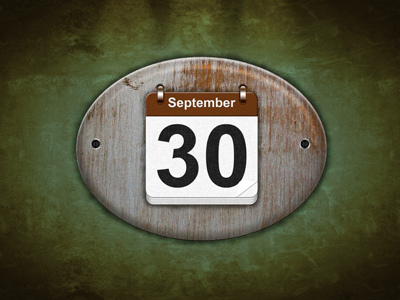30 is a multiple of 5, 6 and 15.

# Multiples (Easy)

As a reminder, a 'multiple' is a number that can be divided exactly by another given number, e.g. 8 is a multiple of 2 because 2 divides 8 exactly four times. By the way, 5.4 is a multiple of 1.8 because 1.8 divides 5.4 exactly three times.

In this 11-plus Maths quiz we shall only be dealing with whole numbers BUT it's a good idea to keep a lookout for multiples when you work with decimals. The times tables that you have learnt will help you in this quiz.

If you haven't already played our first set of very easy quizzes on multiples, take a look before tackling this one. It will get your brain in gear and ready for this slightly harder challenge.

Have fun!

1.
Which of the four choices is a multiple of ALL the numbers given?
5, 6, 15
30
75
36
45
75 isn't divisible by 6, 36 is only divisible by 6 and 45 isn't divisible by 6
2.
Which of the four choices is a multiple of ALL the numbers given?
8, 9, 12
96
72
84
74
96 isn't divisible by 9, 84 is only divisible by 12 and 74 isn't divisible by any of them
3.
Which of the four choices is a multiple of ALL the numbers given?
6, 7, 8
252
42
48
336
A good strategy is as follows: find a likely multiple and check to see if one of the given numbers DOESN'T divide it. If you can't find one, then that's your multiple. If you do find one, then that's NOT your multiple, e.g. 8 doesn't divide 42. TIP! If you see really big likely multiples, try multiplying the given numbers to see if they can form them: 6 × 7 × 8 = 336
4.
Which of the four choices is a multiple of ALL the numbers given?
3, 9, 11
33
27
99
66
33 isn't divisible by 9, 27 isn't divisible by 11 and 66 isn't divisible by 9
5.
Which of the four choices is a multiple of ALL the numbers given?
15, 25, 30
300
230
375
525
230 isn't divisible by any of them. 375 and 525 aren't divisible by 30
6.
Which of the four choices is a multiple of ALL the numbers given?
7, 6, 14
82
48
42
86
82 isn't divisible by any, 48 is only divisible by 6 and 86 isn't divisible by any
7.
Which of the four choices is a multiple of ALL the numbers given?
4, 8, 16, 32
64
36
68
126
36 and 68 are only divisible by 4, and 126 isn't divisible by any of them
8.
Which of the four choices is a multiple of ALL the numbers given?
6, 9, 18
52
27
48
36
52 isn't divisible by any of them, 27 is only divisible by 9 and 48 is only divisible by 6
9.
Which of the four choices is a multiple of ALL the numbers given?
6, 11, 12
132
66
72
121
A good strategy is as follows: find a likely multiple and check to see if one of the given numbers DOESN'T divide it. If you can't find one, then that's your multiple. If you do find one, then that's NOT your multiple, e.g. 12 doesn't divide 66
10.
Which of the four choices is a multiple of ALL the numbers given?
11, 12
121
132
144
111
121 isn't divisible by 12, 144 isn't divisible by 11 and 111 isn't divisible by either of them
Author:  Frank Evans